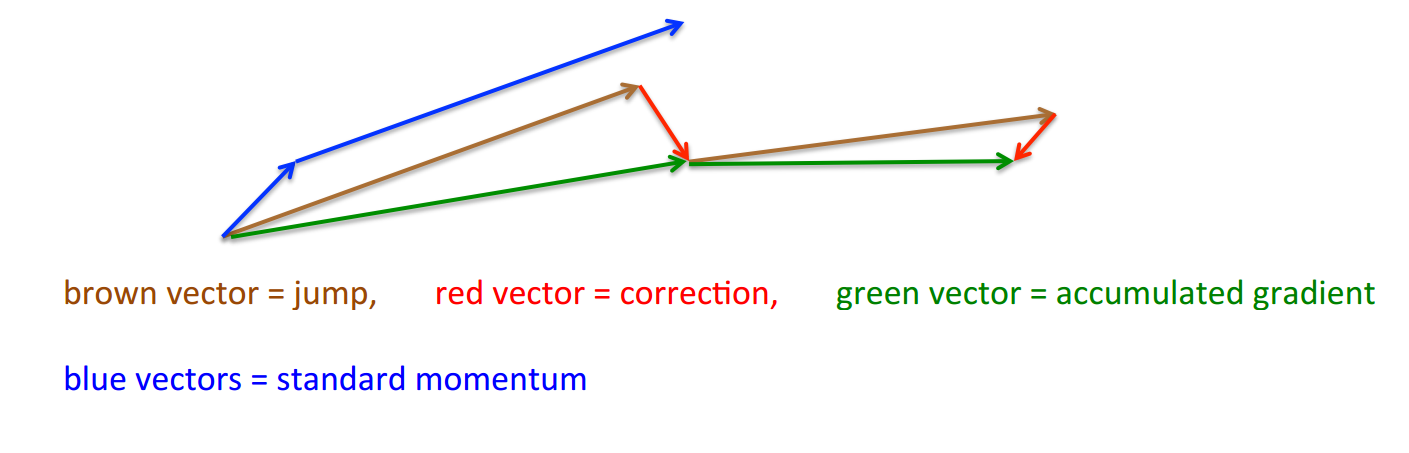# 梯度下降算法优化方法

## 梯度调整相关

### Momentum 动量法

$\gamma$通常设置为0.9或者其他小于1的值，这种方法使得参数在“确认的方向”上前进的更快，而在“犹豫的方向”上减慢下降速度，通常在确认问题有唯一全局最优时非常有效。

### Nesterov 加速梯度Nesterov

## 学习速率相关

Adadelta是Adagrad的一种改进（从名字上也能猜出来），首先在累计梯度平方和的时候，该算法不会将从头开始的梯度一直累加，而是使用衰减平均累加，我们用$E[g^2]$来表示，那么$E[g^2]_t=\gamma E[g^2]_{t-1}+(1-\gamma)g_t^2$

### RMSprop

Adaptive Moment Estimation(Adam)是另一种为每个参数计算不同学习速率的方法，即存储了梯度平方的指数衰减平均（和Adagrad一样），也存储了梯度本身的指数衰减平均：$m_t = \beta_1 m_{t-1} + (1 - \beta_1) g_t$ ,$v_t = \beta_2 v_{t-1} + (1 - \beta_2) g_t^2$ （$m,t$都初始化为0），但是作者发现他们都容易偏移到0，特别是衰减速率较小的情况下（$\beta$很接近1）。

$\hat{m}_t = \frac{m_t}{1 - \beta^t_1}$，
$\hat{v}_t = \frac{v_t}{1 - \beta^t_2}$

## 小结

Nesterov加速度 在动量法基础上先走一段再回正 相较动量法更加“智能” 回正有时适得其反
Adam 使用梯度的一阶和二阶指数衰减平均 收敛速度快，不易陷入局部最小 需要鲁棒的初始化

• 使用全批量梯度下降
• 考虑LBFGS